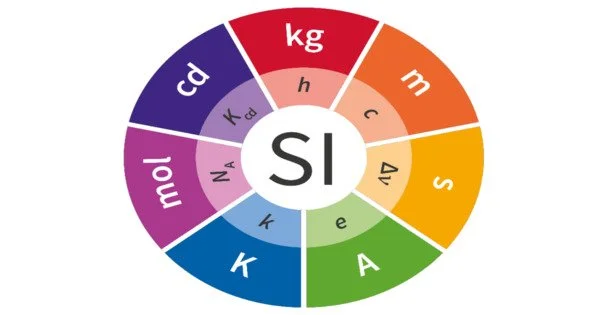Physics

# SI StandardsThe International System of Units (SI) is a global standard for expressing magnitudes or quantities of significant natural phenomena. The System of Units, also known as the metric system, is commonly abbreviated as SI, which comes from the original French name, Système international d’unités.
The SI standard is based on an earlier measurement system known as the meter-kilogram-second (MKS) system.

Length

Length is defined as the distance between two points. The SI unit of length is metre.
One standard metre is equal to 1 650 763.73 wavelengths of the orange − red light emitted by the individual atoms of krypton − 86 in a krypton discharge lamp.

Mass

Mass is the quantity of matter contained in a body. It is independent of temperature and pressure. It does not vary from place to place. The SI unit of mass is kilogram. The kilogram is equal to the mass of the international prototype of the kilogram (a plantinum − iridium alloy cylinder) kept at the International Bureau of Weights and Measures at Sevres, near Paris, France. An atomic standard of mass has not yet been adopted because it is not yet possible to measure masses on an atomic scale with as much
precision as on a macroscopic scale.

Time

Until 1960 the standard of time was based on the mean solar day, the time interval between successive passages of the sun at its highest point across the meridian. It is averaged over an year. In 1967, an
atomic standard was adopted for second, the SI unit of time. One standard second is defined as the time taken for 9 192 631 770 periods of the radiation corresponding to unperturbed transition between hyperfine levels of the ground state of cesium − 133 atom. Atomic clocks are based on this. In atomic clocks, an error of one second occurs only in 5000 years.

Ampere

The ampere is the constant current which, flowing through two straight parallel infinitely long conductors of negligible cross-section, and placed in vacuum 1 m apart, would produce between the conductors a force of 2 × 10 -7 newton per unit length of the conductors.

Kelvin

The Kelvin is the fraction of 1/273.16 of the thermodynamic temperature of the triple point of water.

Mole

The mole is the amount of substance which contains as many elementary entities as there are atoms in 0.012 kg of carbon-12.